Follow

# Scoring: Managing the 'weighting' of checklist score values

Turning on the scoring feature in checklists is a way to fine tune your assessments of learners. What's more, by using the scoring feature in conjunction with a pass/fail threshold, you can specify the minimum value required for a learner to attain a 'successful' completion of the task.

However, though the scoring feature is easy to turn on, and the scores are easy to edit in the answers of your checklist, it is worth considering how the values of each score naturally 'weight' some answers as more valuable than others.

_____

### Why consider the 'weight' of your checklist scores?

The following two options capture one piece of assessment data only. However, the top answer value for the first question is only 1 point, whereas the second question is potentially worth 5 points to the learner. This scoring setup makes the second question five times more valuable to the learner's total score than the first.

Sample Question One...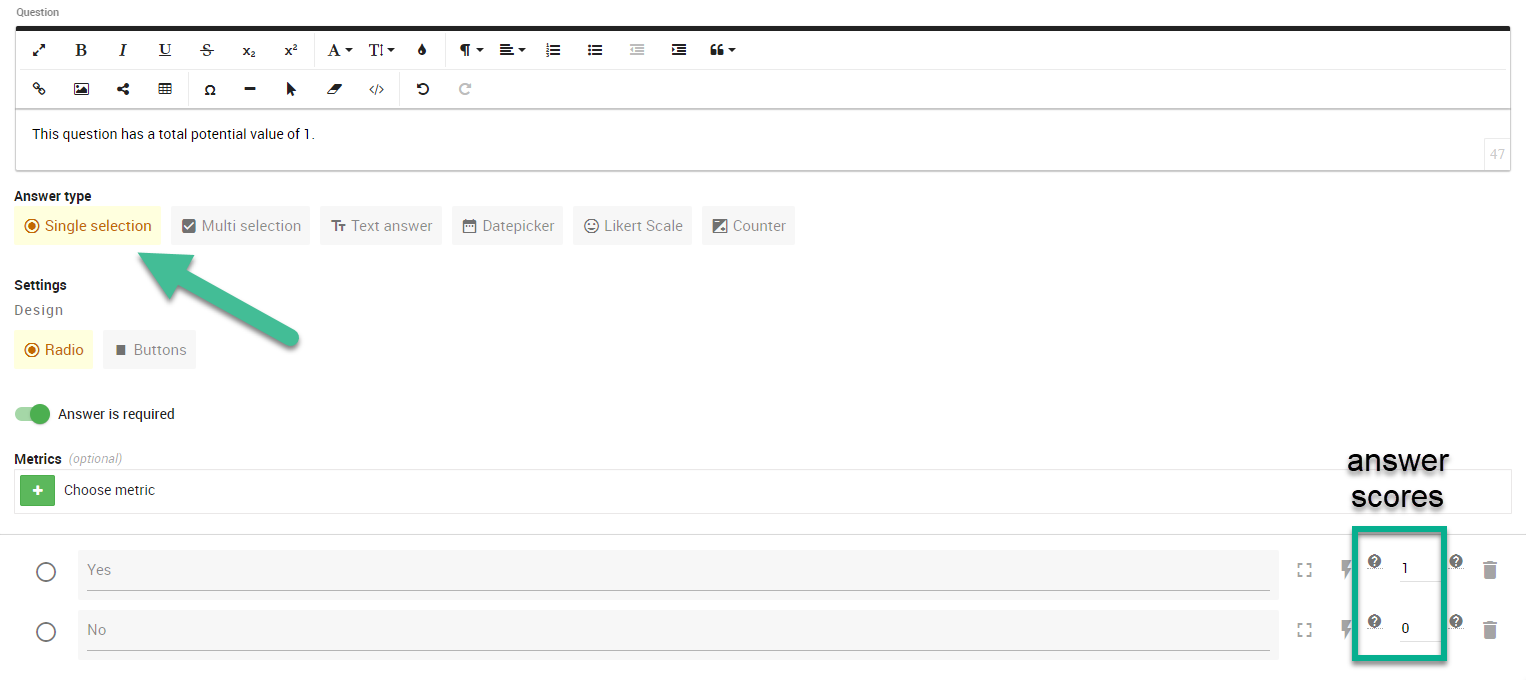Sample Question Two...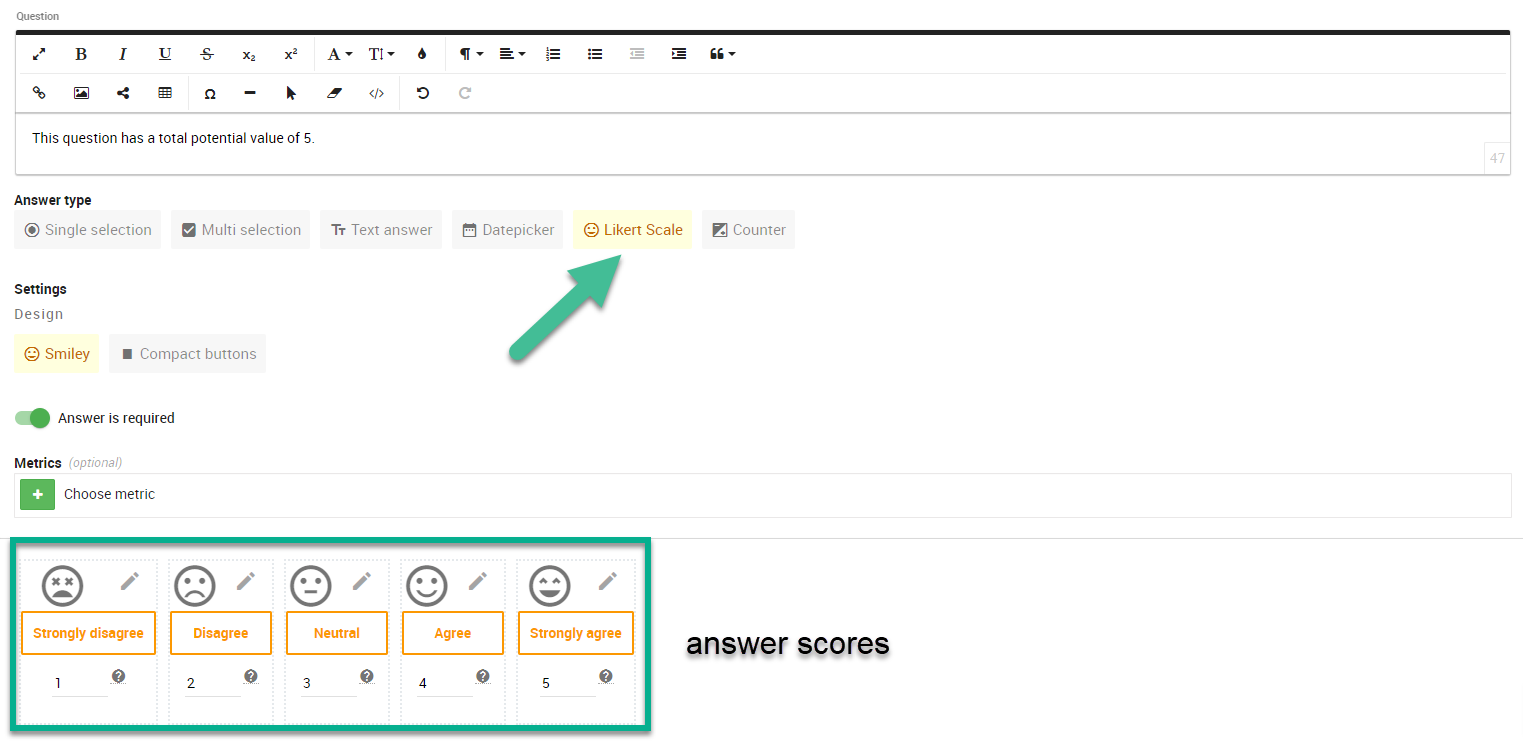it is possible that the data captured by the more 'valuable' questions is indeed more important that the other data captured elsewhere in your checklist, and - in this event - the disparate weighting may not be an issue. This may occur when a hard skill is more essential than others soft skills being assessed in your checklist.

It may also be appropriate to have a higher weight apportioned when you have a multi-select question which captures the demonstration of multiple skills in one answer (see example below).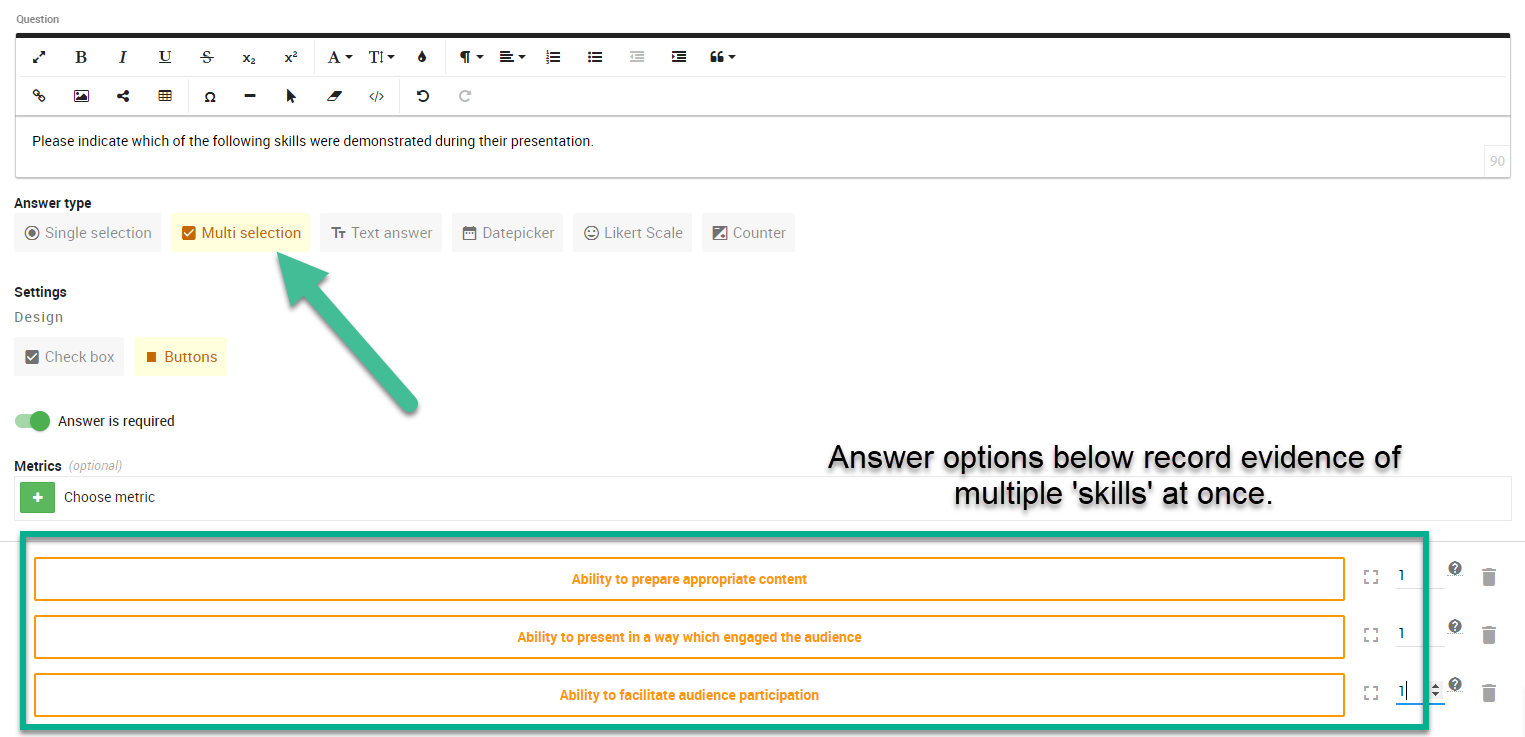In this case, the data captured here is equivalent to three single select options in a checklist assessing the same, and so it having a value three times higher than single select questions in the same checklist is logical.

To prove the reasonable greater value of this question, here are the same assessment criteria presented as three separate questions.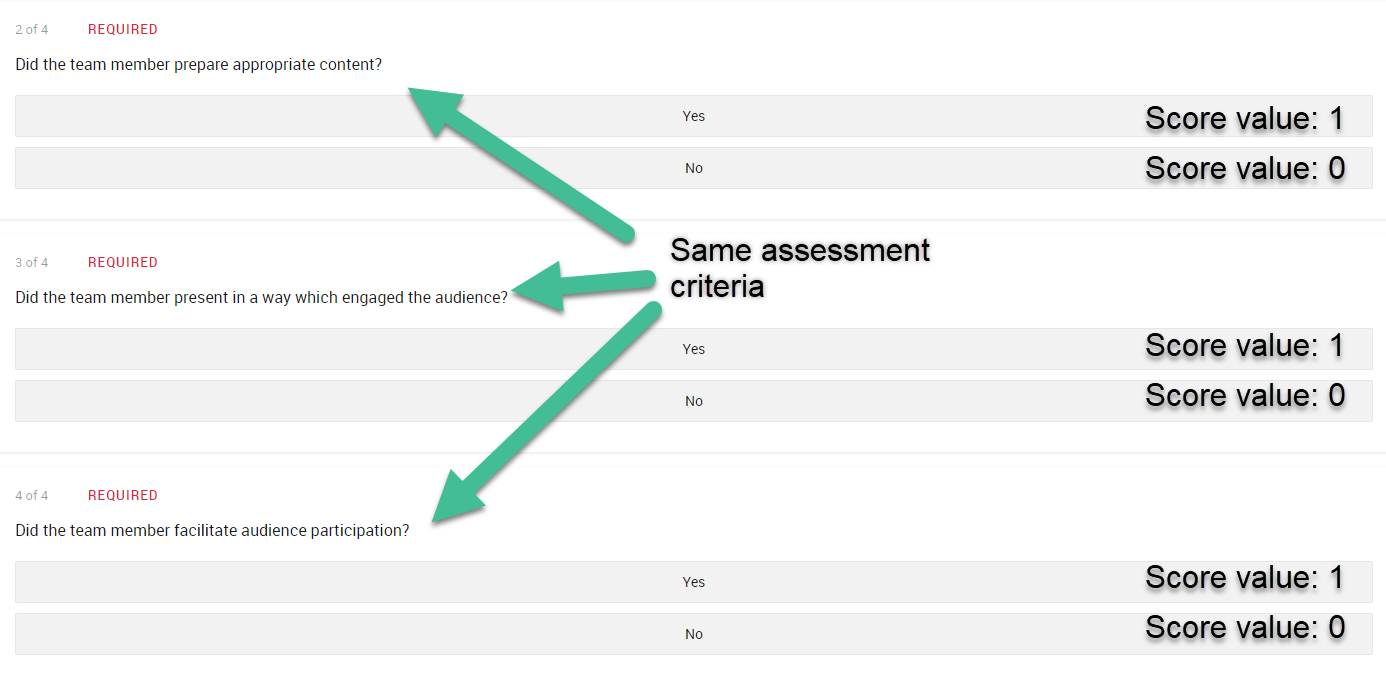If, after considering the intended 'value' of your questions, you decide you'd prefer to more evenly distribute or 'flatten' the weighting of each of your questions, let's look at how this might be managed.

### Flattening score values in your checklist

Let's take the concept from above and apply it to an imaginary checklist to see how we might address this issue.

The example below contains a mix of single select and multi-select answers of varying value.

Imaginary checklist

Q1 = 0 or 1 (single select)
Q2 =  0 or 1 or 2 (single select)
Q3 = 0-10 (single select)
Q4 = 0 or 1 or 2 or 3 (three multi-select answers worth 1 point each)

>> Checklist total possible score = 13

The weight of each question can be presented as a percentage of this total score.
Q1 = 8%
Q2 = 16%
Q3 = 77%
Q4 = 23%

... And we can consider this same information as a graph...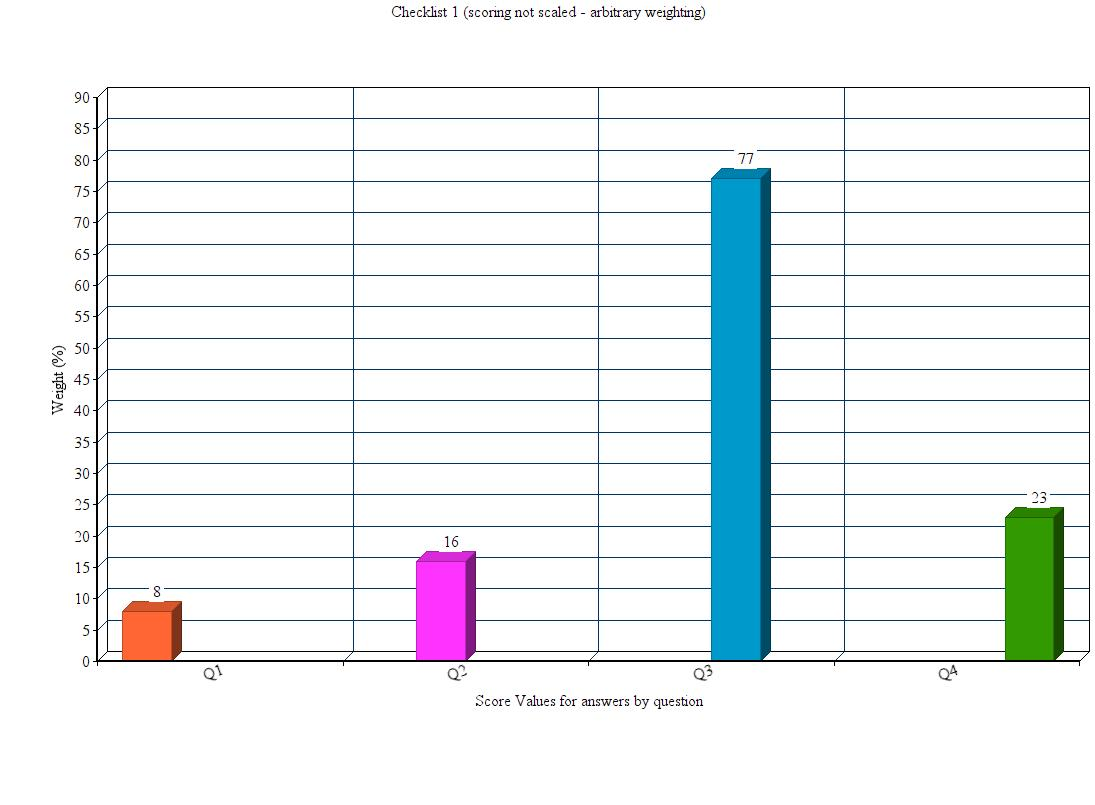It quickly becomes clear in this example that though the multi select question offers three opportunities for a learner to get points, it is the question with an answer scale of 1-10 (i.e. Question 3) which throws out the balance of the overall checklist.

A way to make sure every question has something close to equal weight in overall score is to identify the highest answer score you have in your checklist, and scale everything to this top value.

For example, if there is one question in your checklist in which the max answer value is 10, taking all of the other answer score values and multiplying these by 10 brings your lower answer scores up closer to an equal scale set by your highest checklist score value.

Let's take the original score values from above, and apply this logic to see how the 'weight' of each question re-distributes...

Imaginary checklist v.2 : same values scaled by 10 to flatten the weight of Q3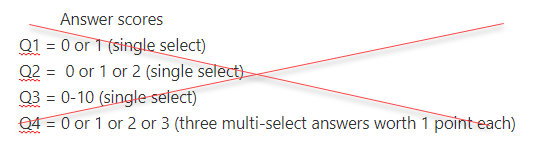Q1 = 0 or 10 (single select)
Q2 = 0 or 10 or 20 (single select)
Q3 = 0-10 (single select) < Note, this one hasn't changed because it's determining the scale for everything else
Q4 = 0 or 10 or 20 or 30 (3 multi-select answers worth 10 each)

>> Checklist total possible score = 70

The weight of each question presented as a percentage of this total score.
Q1 = 14%
Q2 = 28%
Q3 = 14%
Q4 = 43%

... And, the new graph...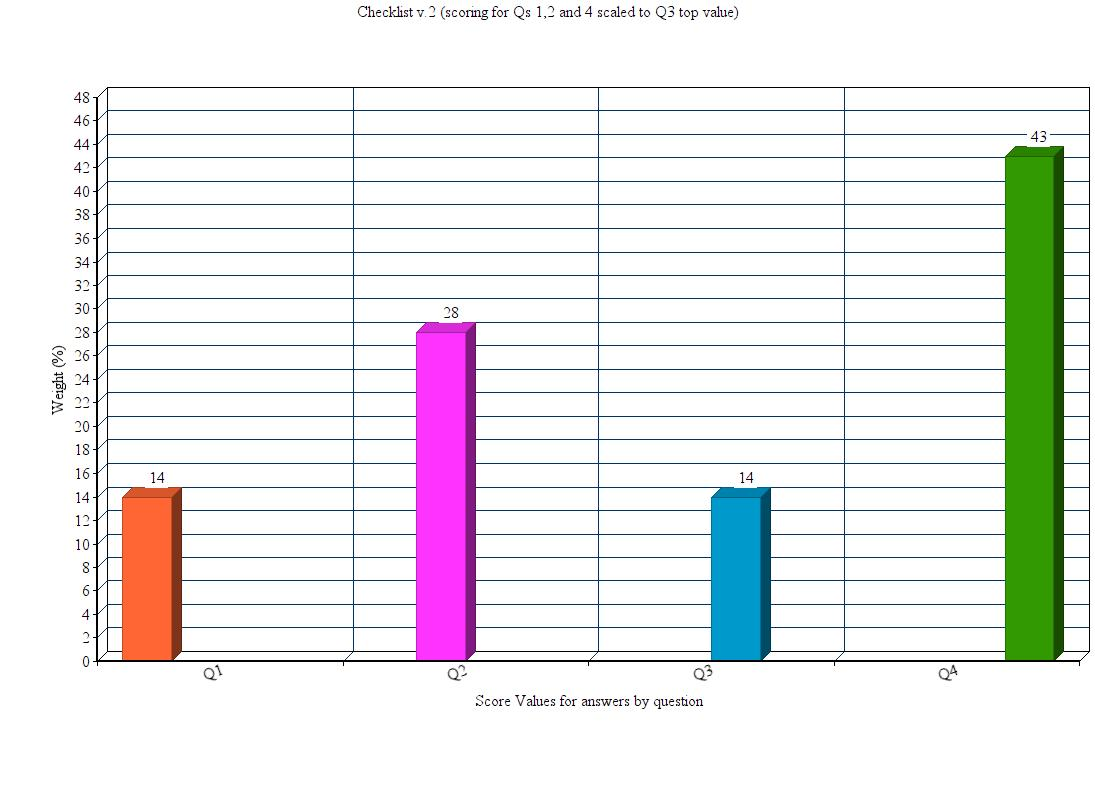The effect this adjustment has on the weighting of Question 3 is apparent - it now has the same overall value as Question 1.

However, Question 2 is now weighted with two times greater value (28% instead of 14%) than Questions 1 and 3. It is possible this is okay and appropriate for your purpose. If it is not, halving the scores to make the answer values 0 or 5 or 10 is an easy way to give Q2 equal weight to Q1 and 3.

Imaginary checklist v.3: Q2 scores changed so that Q2 weight is equal to weight of Qs 1 and 3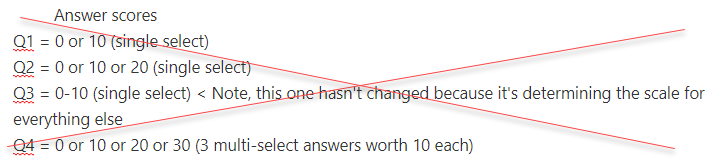Q1 = 0 or 10 (single select)
Q2 = 0 or 5 or 10 (single select)
Q3 = 0-10 (single select)
Q4 = 0 or 10 or 20 or 30 (3 multi-select answers worth 10 each)

>> Checklist total possible score = 60

The weight of each question presented as a percentage of this total score.
Q1 = 16.5%
Q2 = 16.5%
Q3 = 16.5%
Q4 = 51%

... And, a final graph...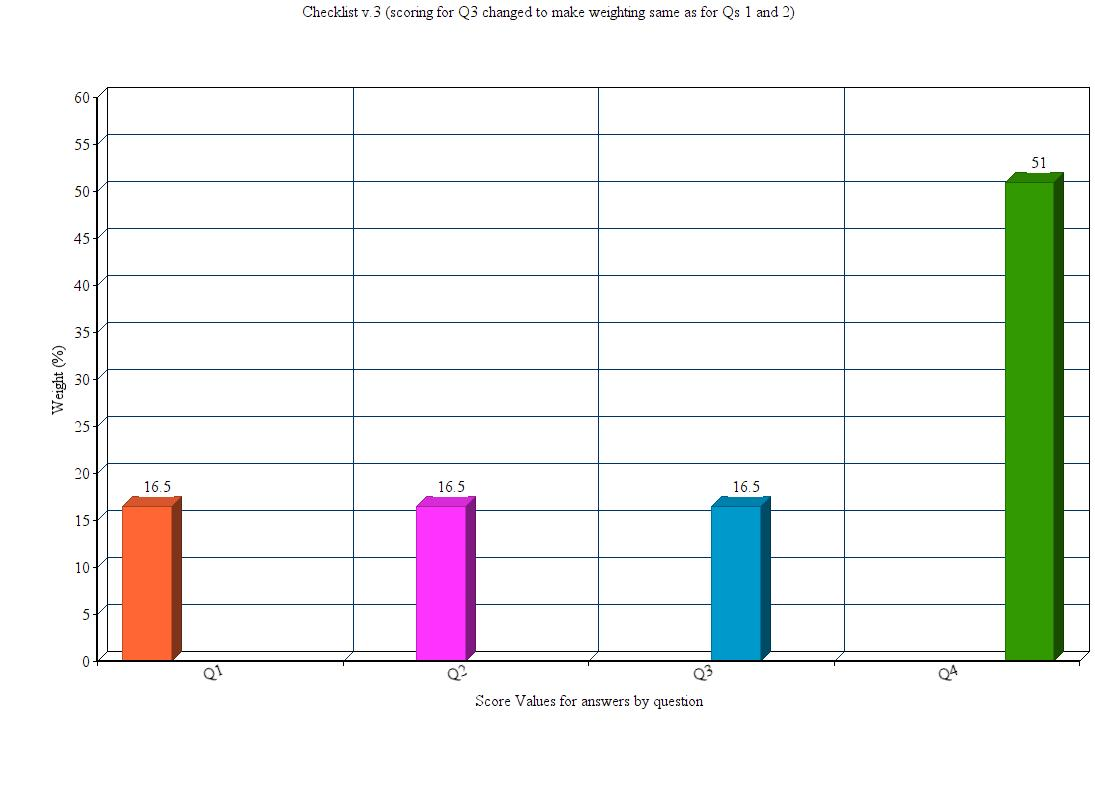We have now achieved equal weighting for Questions 1-3 in this checklist. Question 4 has an obviously greater value. However, as discussed above regarding multi-select question types, it is entirely reasonable that this question retains its greater value due to the number of assessment criteria contained within this score.

Note: These examples assume that all scored questions in a checklist are 'required'. Where checklist results need to be compared across learners, it is often best practice to not make any 'scored' answers optional - this would potentially change the total score for each learner, and means their results might not be comparable.

Article ID: xapimedA_20200320_1